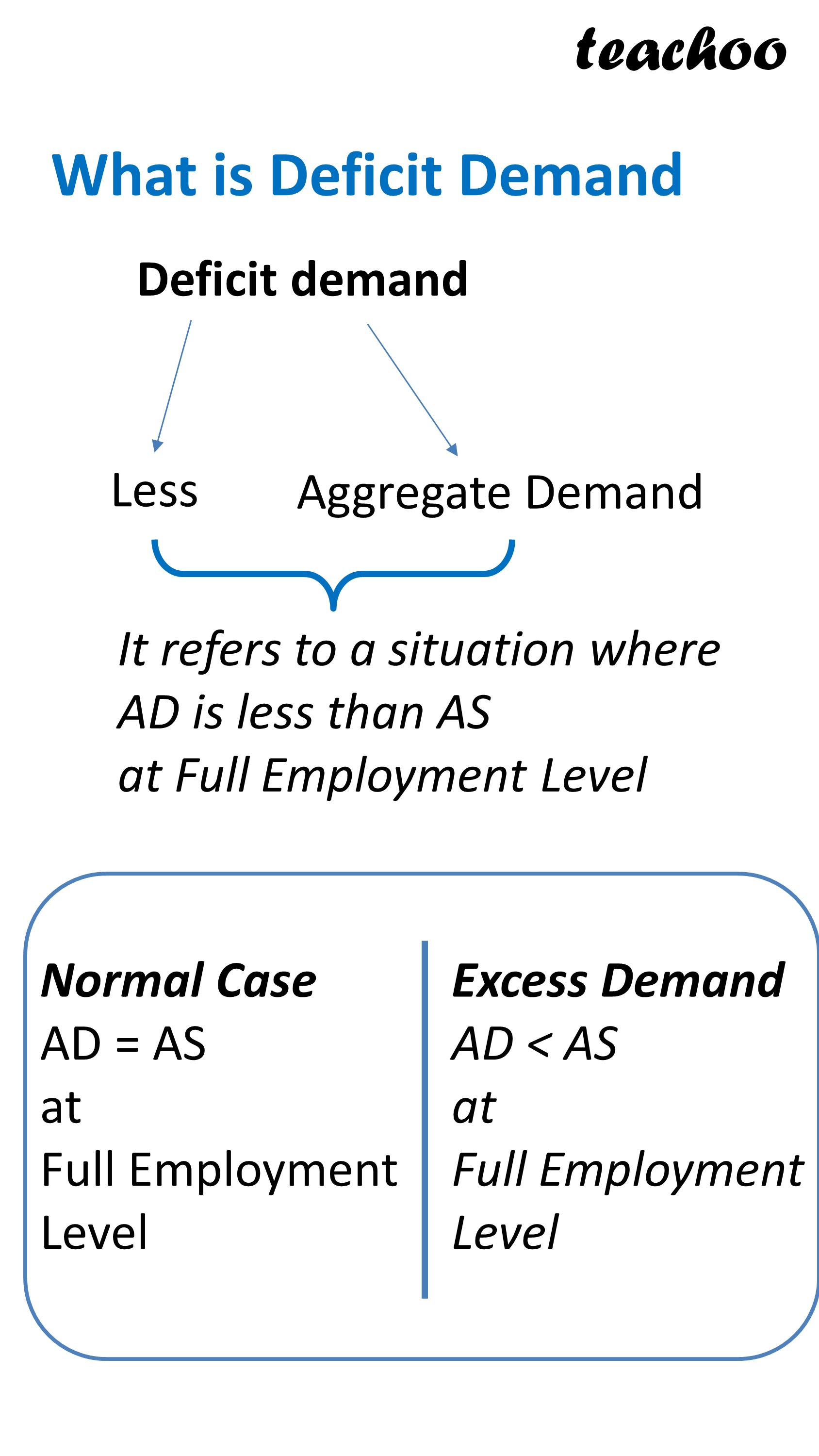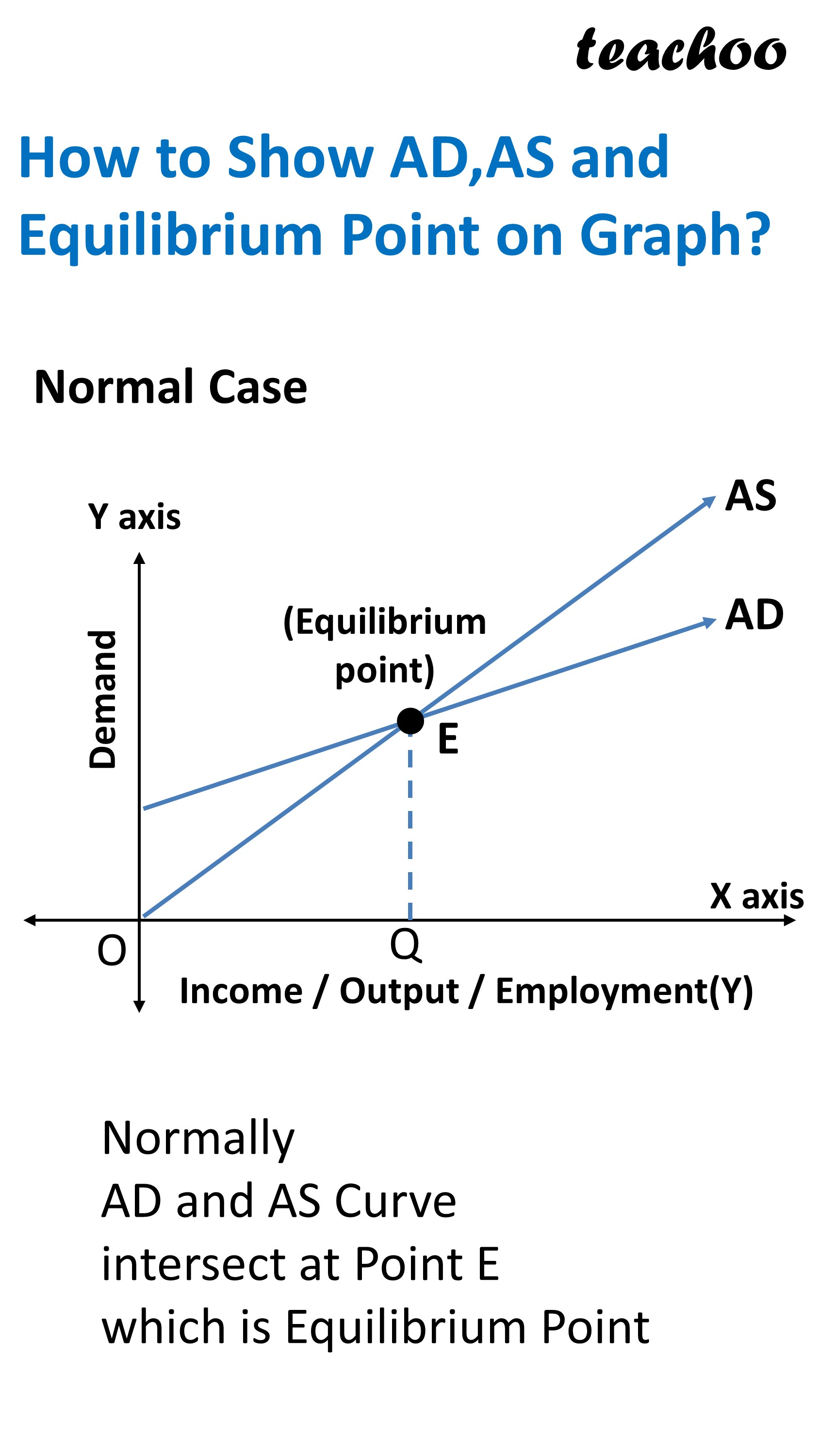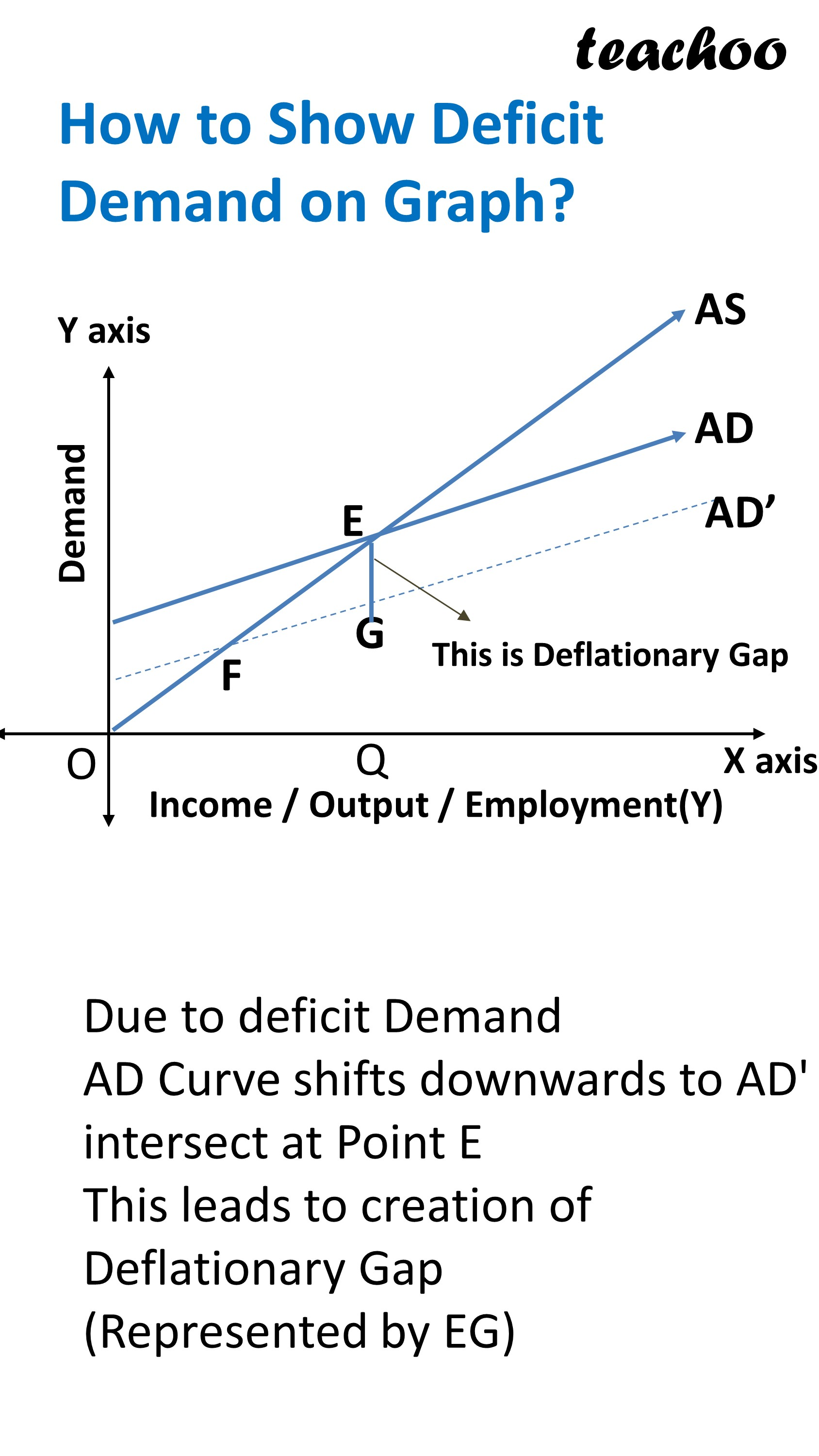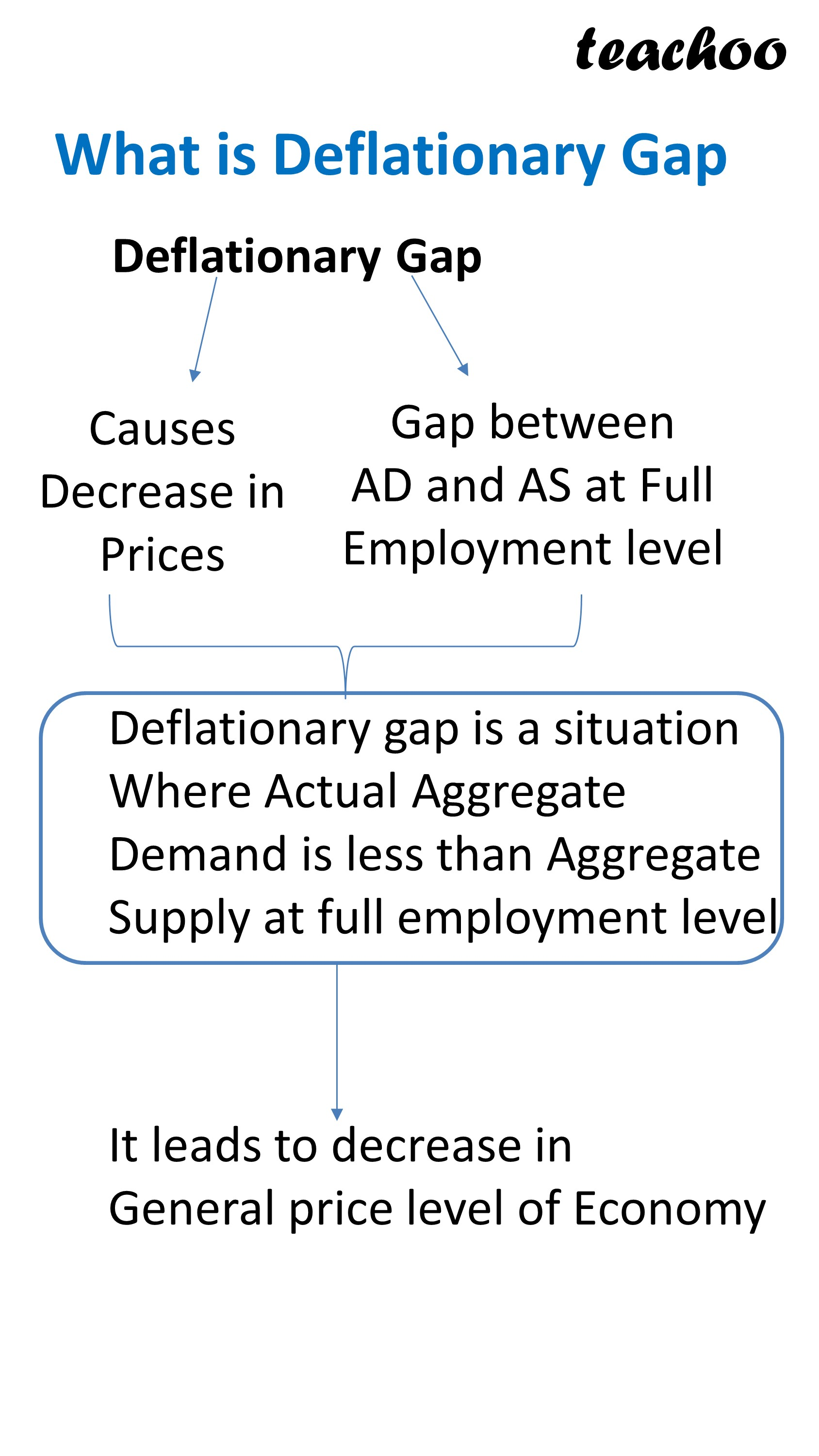Chapter 4 Part 3 - Excess Demand and Excess Supply

Economics Class 12
Macroeconomics

## Deficit Demand

It refers to a situation where

Aggregate Demands is less than Aggregate Supply

at full employment level

Normally

Deficit Demand

AD < AS at equilibrium Level## Note

Deficit Demand leads to Deflationary gap

Deflationary gap is a situation where

Actual Aggregate Demand is less than Aggregate Supply

at full employment level

## Graph Presentation

On X Axis, we represent Income or Output

On Y Axis, we show Demand

It can be seen that

AS Curve is Positively Sloping starting from Origin making an angle of 45 degree with x axis

AD Curve is also starting from Point C

Both AD and AS Curve intersect at Point E which is the equilibrium Point

This leads to creation of Deflationary Gap (Represented by EF)## What is Deflationary Gap?

It is the gap between

Aggregate Demand at full employment level

and

Actual Aggregate Demand

## Effect of Deflationary Gap/Deficient Demand

It leads to decrease in General Price level in an economy

This is because Aggregate Demand decreases but Aggregate Supply remains constantLearn in your speed, with individual attention - Teachoo Maths 1-on-1 Class

### Transcript

What is Deficit Demand Deficit demand Less Aggregate Demand It refers to a situation where AD is less than AS at Full Employment Level Normal Case AD = AS at Full Employment Level Excess Demand AD < AS at Full Employment Level How to Show AD,AS and Equilibrium Point on Graph? Normal Case Normally AD and AS Curve intersect at Point E which is Equilibrium Point How to Show Deficit Demand on Graph? Due to deficit Demand AD Curve shifts downwards to AD' intersect at Point E This leads to creation of Deflationary Gap (Represented by EG) What is Deflationary Gap Deflationary Gap Causes Decrease in Prices Gap between AD and AS at Full Employment level Deflationary gap is a situation Where Actual Aggregate Demand is less than Aggregate Supply at full employment level It leads to decrease in General price level of Economy